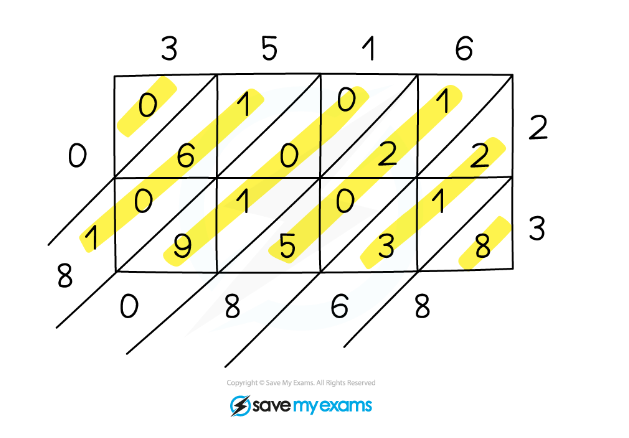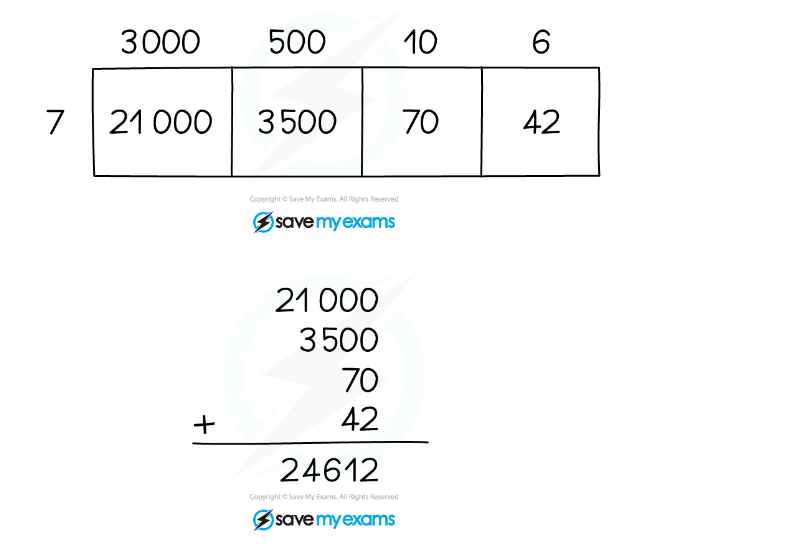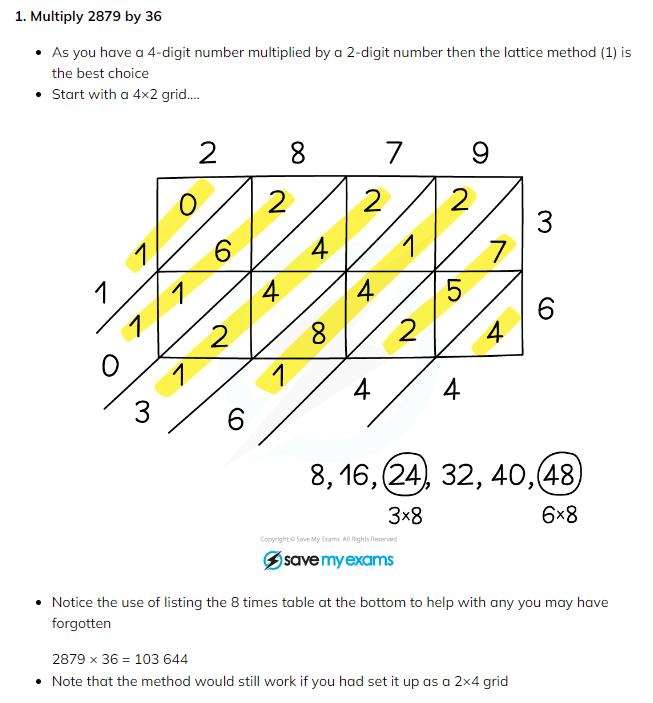# Edexcel IGCSE Maths 复习笔记 1.17.1 Multiplication (non-Calc)

Edexcel IGCSE Maths 复习笔记 1.17.1 Multiplication (non-Calc)

#### (Non-calculator) multiplication – why so many methods?

• Different methods work for different people, and some are better depending on the size of number you are dealing with
• We recommend the following 3 methods depending on the size of number you are dealing with

(If in doubt all methods will work for all numbers!)

#### 1. Lattice metho

(Best for numbers with two or more digits)

• This method allows you to work with digits
• So in the number 3 516 you would only need to work with the digits 3, 5, 1 and 6
• So if you can multiply up to 9x9 you can’t go wrong!So, 3516 × 23 = 80 868

#### 2. Partition method

(Best when one number has just one digit)

• This method keeps the value of the larger number intact
• So with 3 516 you would use 3000, 500, 10 and 6
• This method is not suitable for two larger numbers as you can end up with a lot of zero digits that are hard to keep track ofSo, 3516 × 7 = 24 612

(Best for smaller, simpler cases)

• You may have seen this called ‘chunking’
• It is a way of building up to the answer using simple multiplication facts that can be worked out easily

eg. 13 × 23

1 × 23 = 23

2 × 23 = 464 × 23 = 92

8 × 23 =184

So, 13 × 23 = 1 × 23 + 4 × 23 + 8 × 23 = 23 + 92 + 184 = 299

#### Decimals

• These 3 methods can easily be adapted for use with decimal numbers
• You ignore the decimal point whilst multiplying but put it back in the correct place in order to reach a final answer

eg. 1.3 × 2.3

Ignoring the decimals this is 13 × 23, which from above is 299There are two decimal places in total in the question, so there will be two decimal places in the answer

So, 1.3 × 2.3 = 2.99

#### Exam Tip

If you do forget your times tables then in the exam write a list out of the table you need as you do a question.So for example, if you need to multiply by 8, and you’ve forgotten your 8 times tables, write it down: 8, 16, 24, 32, 40, 48, etc. as far as you need to.

Worked ExampleWorked Example

2. Pencils are sold in boxes. Each box costs £1.25 and each box contains 15 pencils.Tyler buys 35 boxes of pencils.(a) Work out how many pencils Tyler has in total.(b) Work out the total cost for all the boxes Tyler buys.

(a)

This is a roundabout way of asking you to work out 15×35

As this is a simpl-ish case (3) you should use the repeated addition method

1 × 35 = 35

2 × 35 = 70

4 × 35 = 140

8 × 35 = 280

16 × 35 = 560

It doesn’t matter if you go past 15 …

15 × 35 = 16 × 35 – 1 × 35 = 560 – 35

15 × 35 = 525

Worked Example#### Exam Tip

Okay, getting the highlighter out during an exam may be a touch excessive! But do use your grid/diagram to help you answer the question – the highlighter in the example above makes it clear which digits to add up at each stage.  You can do this in pen or pencil but do make sure you can still read the digits underneath as it is all part of your method/working.